# Free vibrations with damping

In the introductory tutorial in this series we considered a free vibrating translational spring and mass system with one degree of freedom with viscous damping as shown in Figure 1 below. Mass m connected to a spring with spring constant k is free to move on a horizontal frictionless plane from the static equilibrium position x = 0.  A dashpot provides a damping force F = c.v where v is velocity of mass m and c the damping coefficient.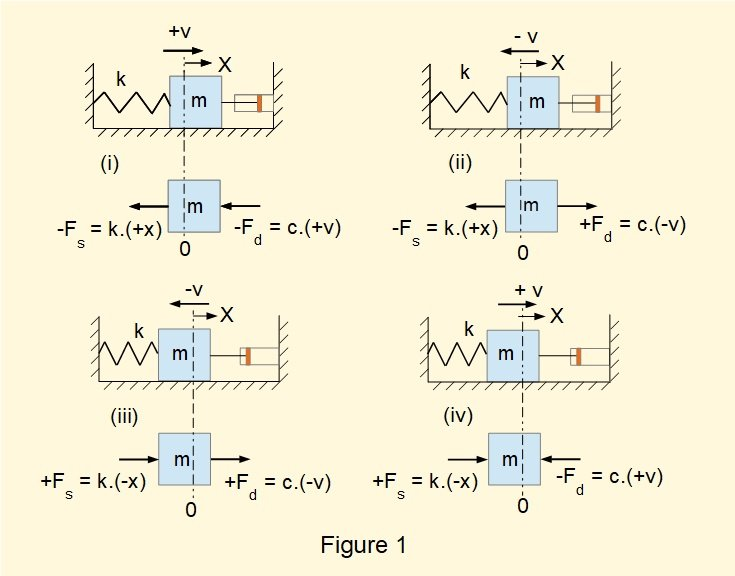From the dynamic* free body diagrams we obtained the following equation of motion for this system:

*  showing forces producing acceleration of mass m, not static equilibrium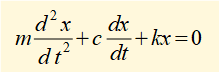We now find a function x(t) that is the general solution to this equation.  If you are not familiar with solving second-order homogeneous linear differential equations using the characteristic equation method refer to the  maths tutorial.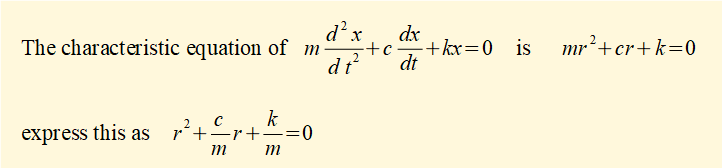In the tutorial on free undamped vibrations we found that k/m = ωn2 where ωn is the natural frequency of the undamped system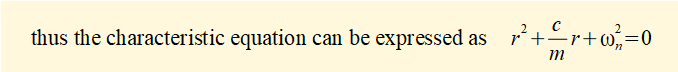Roots r1 and r2 of this equation from the quadratic formula are: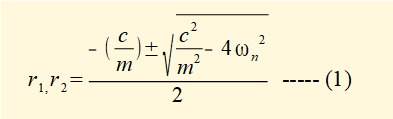We now manipulate this expression as follows to provide a dimensionless factor ζ (zeta) called the damping ratio.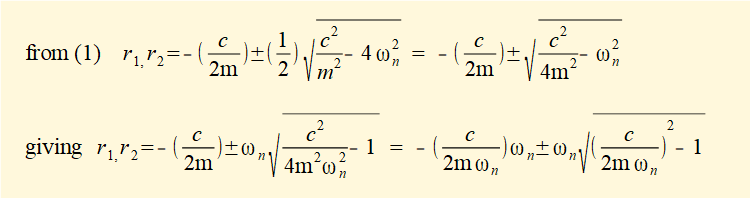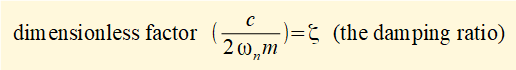(dimensions of the parameters of ζ are: c = M/T   ωn = 1/T   m = M)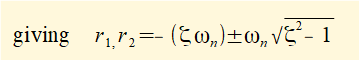From the maths tutorial we know the general solution to the equation of motion using roots r1 and r2 of the characteristic equation is: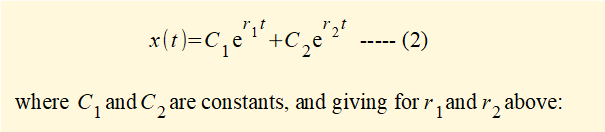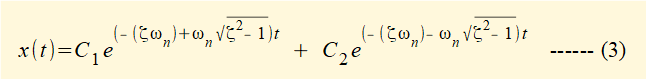We can examine the general forms of this solution for different values of ζ by considering coefficients r1 and r2 of exponent t.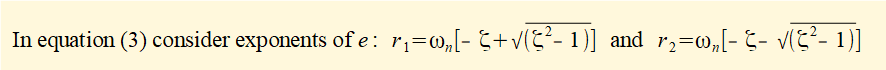#### Where ζ > 1

•  In equation (3) √(ζ 2 - 1) is positive meaning that solutions are real numbers and can be obtained directly from equation (3).
•   √(ζ 2 - 1) < ζ  thus [- ζ  ± √(ζ 2 - 1)] must be negative.  Since ωn  is always positive, both r1 and r2 must be negative.  Therefore the motion must have a decreasing exponential characteristic.

The following example illustrates a damped spring, mass and dashpot system where  ζ > 1 with initial conditions for x(t) = x0 and dx(t)/dt = v0 at time t=0.

To find expressions for constants C1 and C2 in equation (3) we let: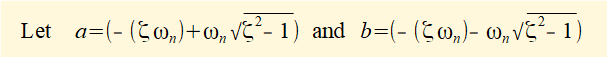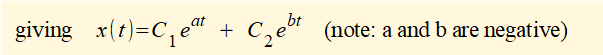At time t = 0:   x0 = C1 + C2   and   v0 = dx/dt = aC1 + bC2       (because e0 = 1)

Solving for C1 and C2 gives: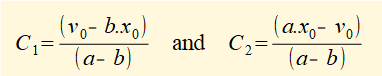Figure 2 below shows a plot of x(t) against time t for a system with the following parameters:

m = 1.0 kg    k = 10 N/m    c = 12 Ns/m     x0 = 0.1 m   v0 = 1.0 m/s   which give ωn = 3.16 rad/s  and  ζ = 1.90

The motion characterised by ζ >1 decreases exponentially with time and is defined as aperiodic.  It is often described as heavily damped.  For comparison the undamped motion of the equivalent free vibrating system derived in the previous tutorial  is also plotted.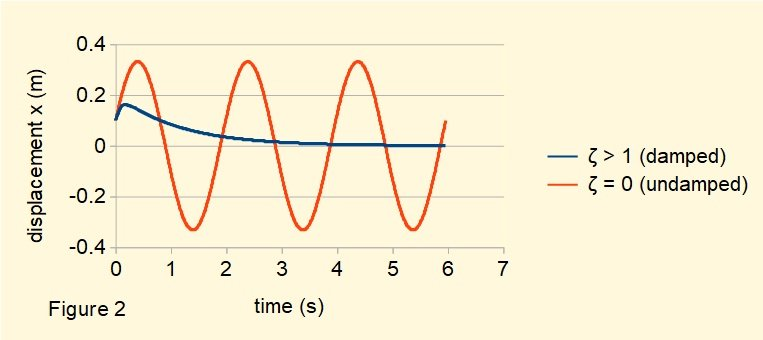#### Where ζ = 1

In equation (3) if  ζ = 1 then  [- ζωn  ±  ωn√(ζ 2 - 1)] = - ωnFrom the maths tutorial we know the general solution to this equation is: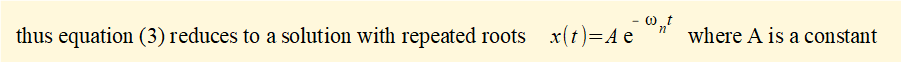To find constants C1 and C2 use initial conditions x0 and v0 at time t = 0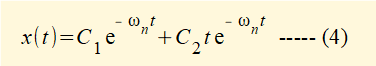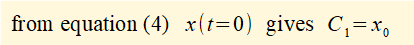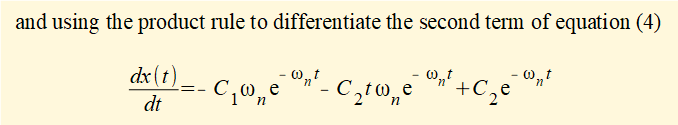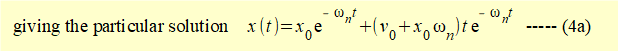Figure 3 below shows a plot for x(t) against time t for the system with  ζ = 1 using the values for m, k and initial conditions in the previous example.  In this case the damping coefficient c = 6.32 Ns/mThe motion characterised by  ζ = 1 is also aperiodic and has the shortest time for decay to the limit  x(t) = 0.  It is called the critically damped condition.

#### Where ζ < 1

Consider roots r1, r2 of the characteristic equation for motion of the system when ζ < 1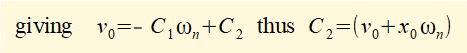If ζ < 1 then  (ζ 2 - 1) is negative and the roots are complex numbers. From the maths tutorial we know the roots are:

r1 = α + i β  and  r2 =  α - i β   where   α = -ωn ζ     and    β = ωn√(1 - ζ 2 )

because   √(ζ 2 - 1)  = √(-1)(1 - ζ 2 )  = i.√(1 - ζ 2 )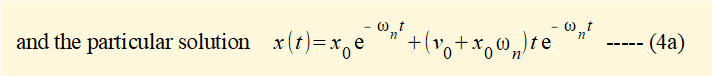To find constants C1 and C2 in equation (5) use initial conditions x0 and v0 at time t = 0

From equation (5)  x(0) = x0 = C1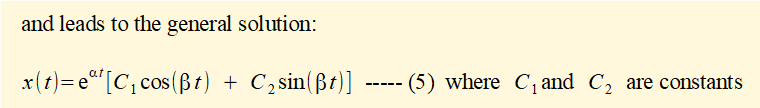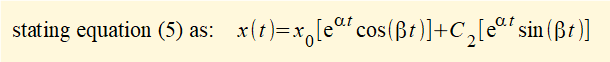which for v0 =  dx/dt at time t = 0 gives: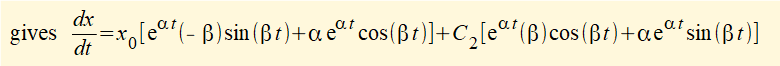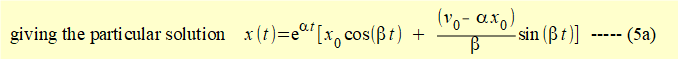Figure 4 below shows a plot of x(t) against time t for the system with  ζ < 1  using the values for m, k and initial conditions in the previous examples   In this case the damping ratio  ζ  = 0.1 and the damping coefficient c = 0.63 Ns/m.When ζ < 1 damping produces a decaying oscillatory motion, often described as light damping.  As it is the most important vibratory behaviour in engineering applications we examine the characteristics in more detail.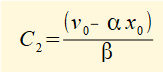From the maths tutorial we know this equation can be expressed as:From above  α = -ωn ζ     and    β = ωn√(1 - ζ 2 )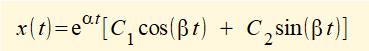Where  A = ((eαtC1)2 +(eαtC2)2)  and  φ = tan-1(eαtC2) / eαtC1

Equation (6) can be interpreted as damped oscillatory motion in two parts.

cos(ωn(√1 - ζ 2 ).t  - φ) expresses harmonic motion at frequency ωn√(1 - ζ 2 ).  We call this the damped natural frequency ωd which differs from the natural frequency of the free vibrating system by the factor √(1 - ζ 2 ).  In most practical applications ζ << 1 and ωd ≈  ωn .  In the example above  ζ  = 0.1 and √(1 - ζ 2 ) = 0.995.  This deviation is impossible to detect on the plot in Figure 4.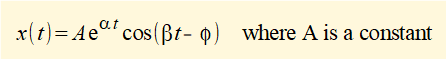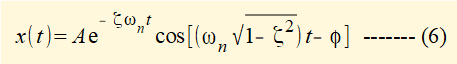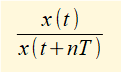We obtained the plot in Figure 5 by assigning a known value to the damping ratio ζ.   It is useful to have an experimental method to find ζ by measuring and plotting the displacement of the system mass against time.  The following method involves measuring successive peaks in the decaying motion.

From Figure 5 the period of each cycle of the decaying harmonic motion is T.  The ratio of the peak displacements  between n cycles can be expressed as;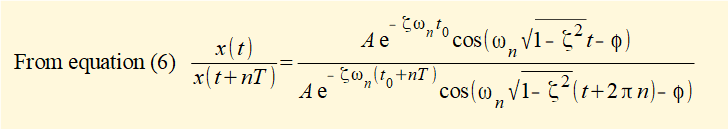Constant A and the cosine terms cancel giving: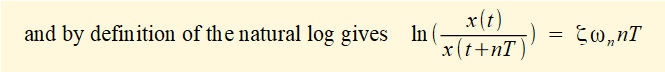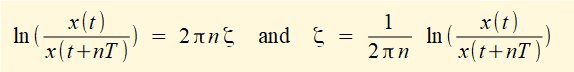Typically  ζ << 1 thus √(1 - ζ 2) ≈ 1 giving: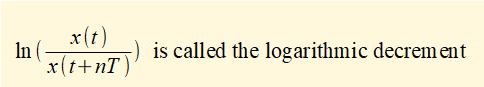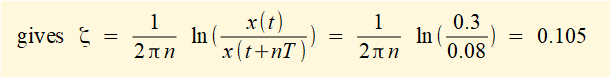We can estimate ζ for the system in the example above from the plot in Figure 5 using peaks x1 and x3 for which n = 2

x1 ≈ 0.30 m     x3 ≈ 0.08 m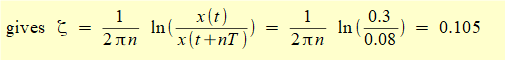Compare with the value assigned for the example  ζ = 0.1

This has been quite a meaty tutorial but if you take the maths slowly step by step to their conclusions interpreting the outcomes is rewarding.

In the next tutorial we consider forced vibration without damping

.

I welcome feedback at:

### Tutorials - mechanical vibrations

###### Forced vibrations with damping

Alistair's tutorials 2021